In this article I am going to explain a use of union operator in SQL Server Database with a real life scenario and example.

The UNION operator is used to combine the result-set of two or more SELECT statements.

Notice that each SELECT statement with the UNOIN must have the same number of columns. The columns must also have similar data types. Also, the columns in each SELECT statement must be in the same order.

Before moving towards union query, let understand what is our scenario. Suppose we have three tables (Product, Customer and Order) in our database and we want to see the number of orders by month name, week days and between three hours gaps in day.

Let’s move towards our example:

1-Product table structure and data. This table is not necessary to create for this example if you already have a ProductId in other table, to relate with Order table.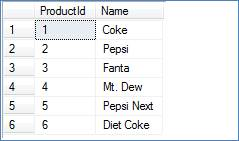2-Customer table structure and data. This table is also not necessary to create for this example if you already have a CustomerId in other table, to relate with Order table.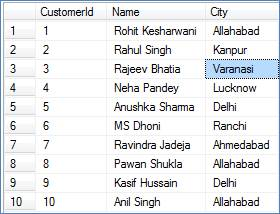3-Order table structure and data. This table is necessary specially OrderDate column of this table because we are going to use OrderDate column in all our further queries which shows us use of union operator in real scenario.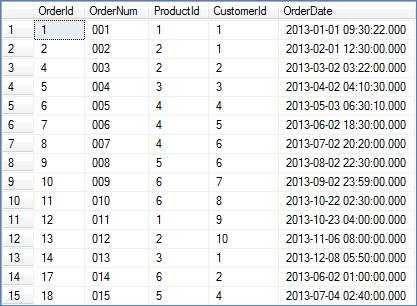4-First union query which show the orders (number of orders received by month) result by month name:

Select [Month], [Orders] From (

Select 'January' as [Month], 1 as [MonthNum], COUNT(*) as [Orders] From [Order] Where MONTH(OrderDate) = 1
Union
Select 'Febuary' as [Month], 2 as [MonthNum], COUNT(*) as [Orders] From [Order] Where MONTH(OrderDate) = 2
Union
Select 'March' as [Month], 3 as [MonthNum], COUNT(*) as [Orders] From [Order] Where MONTH(OrderDate) = 3
Union
Select 'April' as [Month], 4 as [MonthNum], COUNT(*) as [Orders] From [Order] Where MONTH(OrderDate) = 4
Union
Select 'May' as [Month], 5 as [MonthNum], COUNT(*) as [Orders] From [Order] Where MONTH(OrderDate) = 5
Union
Select 'June' as [Month], 6 as [MonthNum], COUNT(*) as [Orders] From [Order] Where MONTH(OrderDate) = 6
Union
Select 'July' as [Month], 7 as [MonthNum], COUNT(*) as [Orders] From [Order] Where MONTH(OrderDate) = 7
Union
Select 'August' as [Month], 8 as [MonthNum], COUNT(*) as [Orders] From [Order] Where MONTH(OrderDate) = 8
Union
Select 'September' as [Month], 9 as [MonthNum], COUNT(*) as [Orders] From [Order] Where MONTH(OrderDate) = 9
Union
Select 'October' as [Month], 10 as [MonthNum], COUNT(*) as [Orders] From [Order] Where MONTH(OrderDate) = 10
Union
Select 'November' as [Month], 11 as [MonthNum], COUNT(*) as [Orders] From [Order] Where MONTH(OrderDate) = 11
Union
Select 'December' as [Month], 12 as [MonthNum], COUNT(*) as [Orders] From [Order] Where MONTH(OrderDate) = 12
) Results Order by MonthNum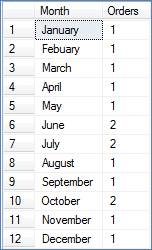Second union query which show the orders (number of orders received by week day) result by week day:

Select [DayofWeek], [Orders] From (

Select 'Sunday' as [DayofWeek], 1 as [WeekNum], COUNT(*) as [Orders] From [Order] Where DATEPART(dw, OrderDate) = 1
Union
Select 'Monday' as [DayofWeek], 2 as [WeekNum], COUNT(*) as [Orders] From [Order] Where DATEPART(dw, OrderDate) = 2
Union
Select 'Tuesday' as [DayofWeek], 3 as [WeekNum], COUNT(*) as [Orders] From [Order] Where DATEPART(dw, OrderDate) = 3
Union
Select 'Wednesday' as [DayofWeek], 4 as [WeekNum], COUNT(*) as [Orders] From [Order] Where DATEPART(dw, OrderDate) = 4
Union
Select 'Thursday' as [DayofWeek], 5 as [WeekNum], COUNT(*) as [Orders] From [Order] Where DATEPART(dw, OrderDate) = 5
Union
Select 'Friday' as [DayofWeek], 6 as [WeekNum], COUNT(*) as [Orders] From [Order] Where DATEPART(dw, OrderDate) = 6
Union
Select 'Saturday' as [DayofWeek], 7 as [WeekNum], COUNT(*) as [Orders] From [Order] Where DATEPART(dw, OrderDate) = 7
) Results Order by [WeekNum]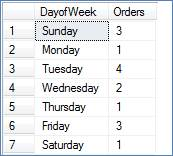Third union query which show the orders (number of orders received by gap of 3 hours in a day) result by gap of 3 hours in a day:

Select [TimeofDay], [Orders] From (

Select '12 AM to 03 AM' as [TimeofDay], 1 as [TimeNum], COUNT(*) as [Orders] From [Order] Where cast(OrderDate as time) between '00:00:00' and '03:00:00'
Union
Select '03 AM to 06 AM' as [TimeofDay], 2 as [TimeNum], COUNT(*) as [Orders] From [Order] Where cast(OrderDate as time) between '03:00:00' and '06:00:00'
Union
Select '06 AM to 09 AM' as [TimeofDay], 3 as [TimeNum], COUNT(*) as [Orders] From [Order] Where cast(OrderDate as time) between '06:00:00' and '09:00:00'
Union
Select '09 AM to 12 PM' as [TimeofDay], 4 as [TimeNum], COUNT(*) as [Orders] From [Order] Where cast(OrderDate as time) between '09:00:00' and '12:00:00'
Union
Select '12 PM to 03 PM' as [TimeofDay], 5 as [TimeNum], COUNT(*) as [Orders] From [Order] Where cast(OrderDate as time) between '12:00:00' and '15:00:00'
Union
Select '03 PM to 06 PM' as [TimeofDay], 6 as [TimeNum], COUNT(*) as [Orders] From [Order] Where cast(OrderDate as time) between '15:00:00' and '18:00:00'
Union
Select '06 PM to 09 PM' as [TimeofDay], 7 as [TimeNum], COUNT(*) as [Orders] From [Order] Where cast(OrderDate as time) between '18:00:00' and '21:00:00'
Union
Select '09 PM to 12 AM' as [TimeofDay], 8 as [TimeNum], COUNT(*) as [Orders] From [Order] Where cast(OrderDate as time) between '21:00:00' and '00:00:00'
) Results Order by [TimeNum]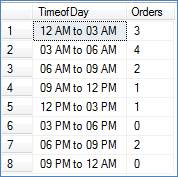Thanks for reading this article. Please suggest me how can I improve this one and other alternative way of doing the similar scenario.

Modified On Sep-07-2019 12:15:01 AM